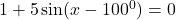## Solve for x: 0⁰ < x< 360⁰​

Question

Solve for x: 0⁰ < x< 360⁰in progress 0
1 week 2021-07-21T22:55:23+00:00 1 Answers 1 views 0

x = 88.463°

Step-by-step explanation:

We are given a trigonometric equation and we need to find out the value of x. The angle x lies between 0° and 360° . That is ,Given trigonometric equation :Subtracting 1 both sides , we have ,Divide both sides by 5 ,This equation can be written as ,